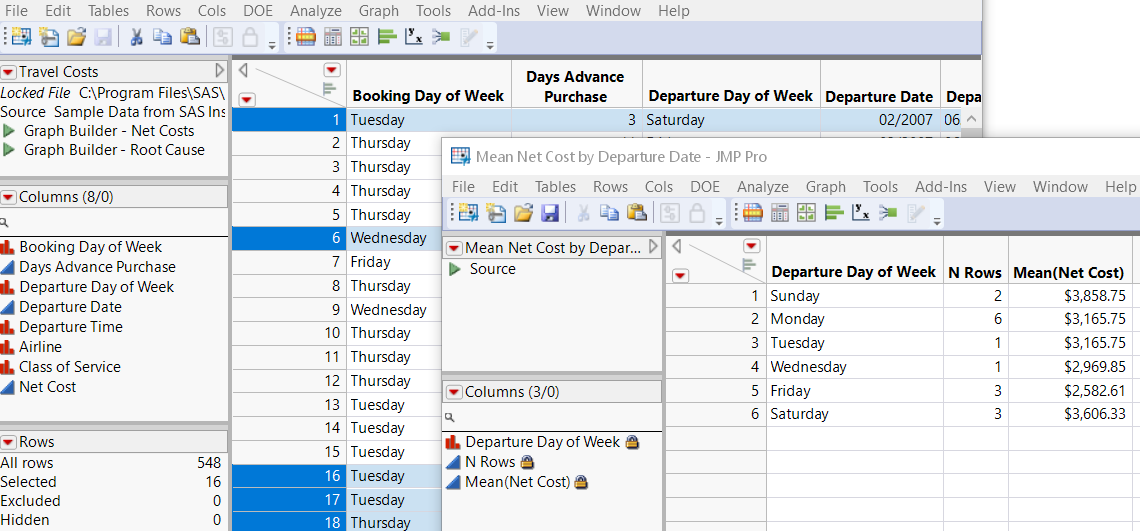Scripting Guide > Common Tasks > Format Date-Time Values and Subset Data
Publication date: 11/10/2021

# Format Date-Time Values and Subset Data

How can you work with dates in JMP? JMP provides a number of formats that you can use to make comparisons and then subset data based on the date.

Select Where Using Dates.jsl applies the Date MDY format to a column of departure dates and subsets the data. A summary table of mean net costs by departure date then appears (Figure 17.2).

`/*  How can you work with dates in JSL? JMP provides a number of formats`
`that you can use to make comparisons and then subset data based on the date.`
`*/`
` `
`hdt = Open( "\$SAMPLE_DATA/Travel Costs.jmp" );`
` `

/* Apply the Date MDY format to Departure Date values and then select only

February dates. */

`hdt << Select Where(`
`		(Date MDY( 02, 01, 2007 ) <= :Departure Date < Date MDY( 03, 1, 2007 ))`
`		);`
` `

/* Subset the selected rows into two tables: one table contains February

departure dates, the other contains all data for those departure dates. */

`nt1 = hdt << Subset( Columns( :Departure Date ),`
`	Output Table Name( "February Departure Date" ) );`
`nt2 = hdt << Subset( Output Table Name( "February Data" ) );`
` `

/* Create a summary table, grouping mean cost by day of week that departure

took place. */

`sumDt = nt2 << Summary(`
`	Group( :Departure Day of Week ),`
`	Mean( :Net Cost ),`
`	Output Table Name( "Mean Net Cost by Departure Date" )`
`);`

Figure 17.2 The Original Table and the Final Summary Table